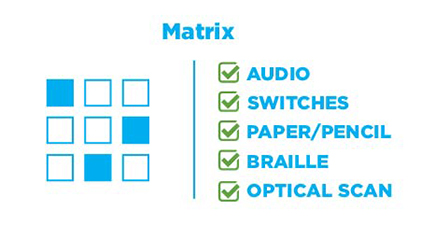# Matrix Labeling

Matrix labeling items contain a grid in which elements are in rows, and the labels are column headers. Depending on the item, labels may be used more than once. In braille, elements are numbered, and there are letters at the intersections of rows and columns to simplify responding with a braillewriter. Users enter a number for each element and a letter to indicate its label.1. Select the x value that makes each equation true.
2.  x = -3 x = -2 x = -4 x = -1 x2 – x – 6 = 0 A B C D 3x2 – 12x – 15 = 0 A B C D 6x2 – 6x – 72 = 0 A B C D 6x2 + 18x – 24 = 0 A B C D